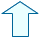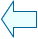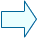THE ANGLES OF A POLYGON Section: Geometry

 1. THE ANGLES OF A POLYGON In this unit we are going to focus on regular polygons as they have the most interesting properties. Furthermore, irregular polygons can be divided into triangles and each triangle can be examined separately. Therefore, we can analyse irregular polygons if we know enough about triangles . 1.- Use the mouse to drag the red points of the closed polygon figure and place them clockwise onto the blue points. You will see that you get a hexagon.2.- Repeat the process but this time place the red points anticlockwise onto the blue points 3.- Notice how different polygons are formed as you move the points (as long as the segments don't cross each other). Some of the polygons formed are convex (all of their vertices point outwards) and others are  not convex (some of their vertices point inwards) and all of the polygons, except the last one formed, are irregular.

2. THE CENTRAL ANGLE OF A REGULAR POLYGON
The following shape is a pentagon in which we have drawn the central angles (the angle formed by two adjacent vertices and the centre of the shape).
1.- Rotate the polygon until two adjacent vertices pass through point P.

2.- Note that the size of the central angle is the difference in value of the angle of rotation of the two points.

 Look carefully at the rotation button to work out the difference in value. The Init button will restore the initial values.

3.- Prove that the central angle in a regular pentagon measures 360/5

4.- Prove that the central angle would measure 360/7 if the polygon had 7 sides.

5.- Does this formula work for squares and triangles? Try it and see. They are polygons too!

 3. THE INTERIOR AND EXTERIOR ANGLES OF A POLYGON Two more angles are illustrated in the following figure: an exterior angle (formed by one side and the extension of the adjacent side) and an interior angle (formed by two adjacent sides). 1.- Notice that both of these angles in the diagram are supplementary angles (i.e. they both add up to 180º). 2.- To work out the size of an exterior angle imagine that you are driving a car around the outside of the polygon. While you are driving along a side the steering wheel is straight but when you get to a vertex you have to move the steering wheel (angle M) to line up the car for the next side (unless you want to drive off the road!) You have to repeat this process 5 times to get back to the starting point again (going in the same direction). We have gone around the shape completely, i.e. 360º and we get the following: M=360/5 3.- Note that the size of the exterior angle is the same as the size of the central angle.Agustín Muñoz NúñezSpanish Ministry of Education. Year 2001